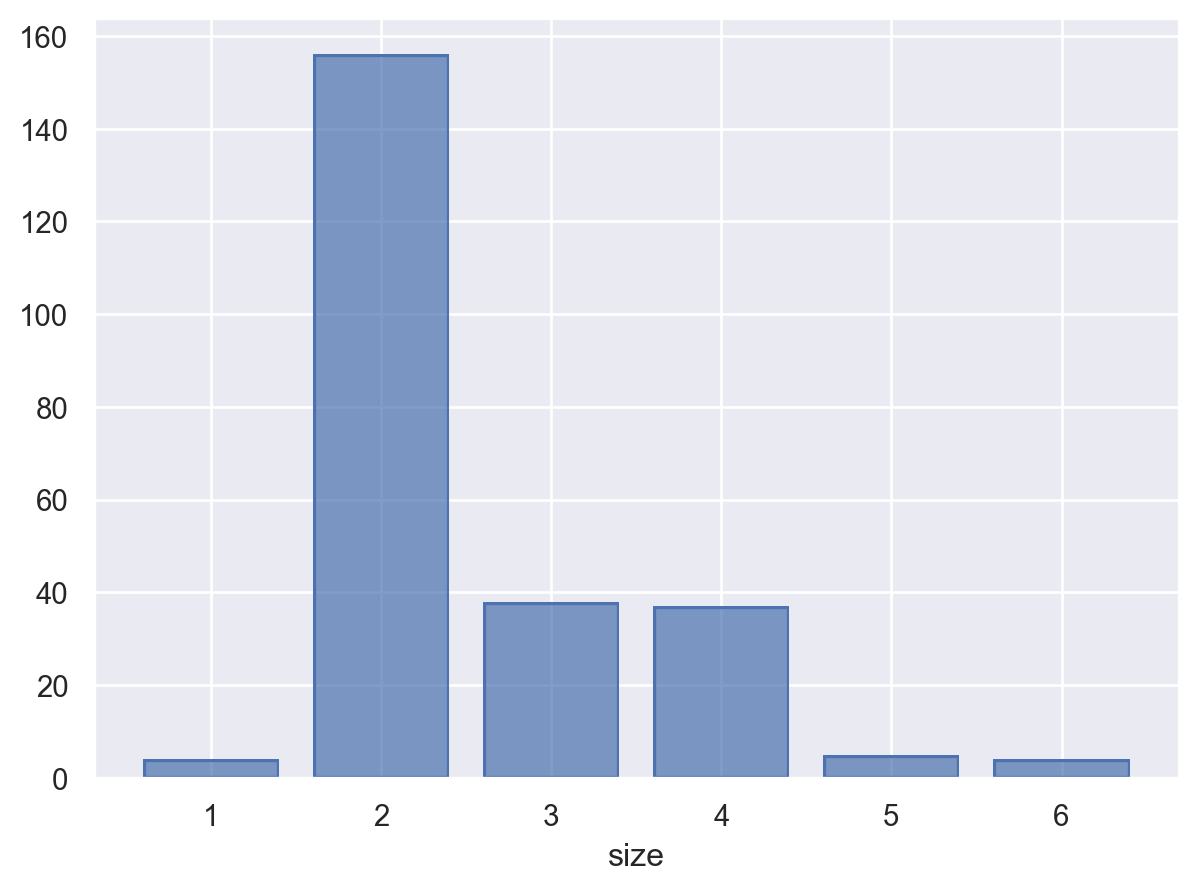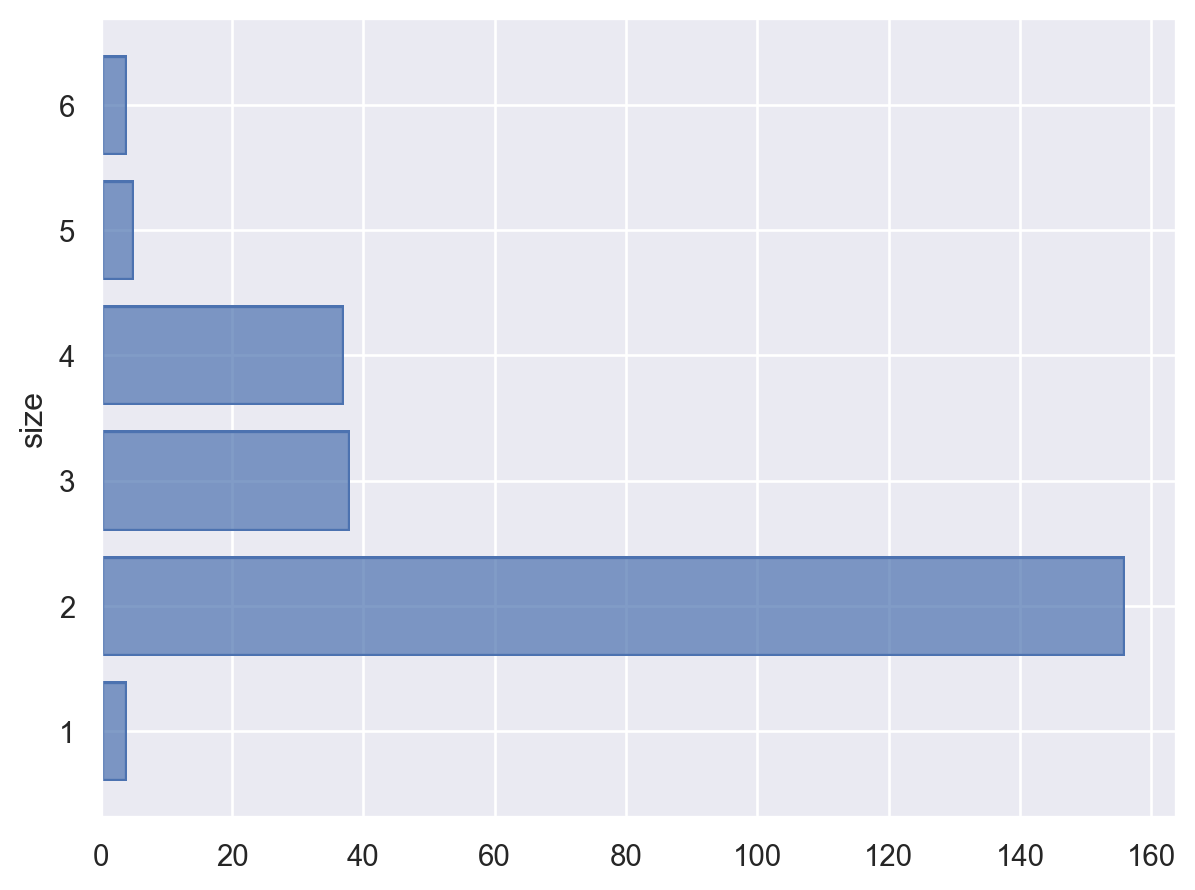# seaborn.objects.Count#

class seaborn.objects.Count#

Count distinct observations within groups.

`Hist`

A more fully-featured transform including binning and/or normalization.

Examples

The transform counts distinct observations of the orientation variable defines a new variable on the opposite axis:

```so.Plot(tips, x="day").add(so.Bar(), so.Count())
```When additional mapping variables are defined, they are also used to define groups:

```so.Plot(tips, x="day", color="sex").add(so.Bar(), so.Count(), so.Dodge())
```Unlike `Hist`, numeric data are not binned before counting:

```so.Plot(tips, x="size").add(so.Bar(), so.Count())
```When the `y` variable is defined, the counts are assigned to the `x` variable:

```so.Plot(tips, y="size").add(so.Bar(), so.Count())
```